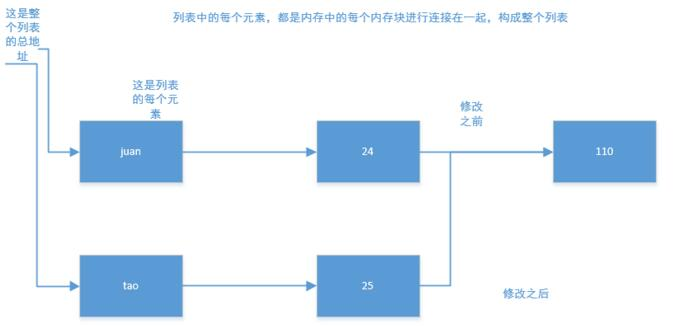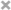# Hunter Yuan

``````a = 2
print id(a)
a = 3
print id(a)
``````

``````31095184
31095160
``````

``````print('Research on the variability of tuple ')
tuple1 = ("juan", 24, "110")
print tuple1

tuple1 = 25
print tuple1
``````

``````TypeError: 'tuple' object does not support item assignment
````````````print('Research on the variability of lists')
list1 = ["juan", 24, "110"]
print list1
print "ID-list1=", id(list1)
print "ID-list1=", id(list1)
print "ID-list1=", id(list1)

list1 = "tao"
list1 = 26
print list1
print "ID-list1=", id(list1)
print "ID-list1=", id(list1)
print "ID-list1=", id(list1)
``````

``````Research on the variability of lists
['juan', 24, '110']
ID-list1= 38301128
ID-list1= 38951672
ID-list1= 31094656
['tao', 26, '110']
ID-list1= 38301128
ID-list1= 38951872
ID-list1= 31094608
``````